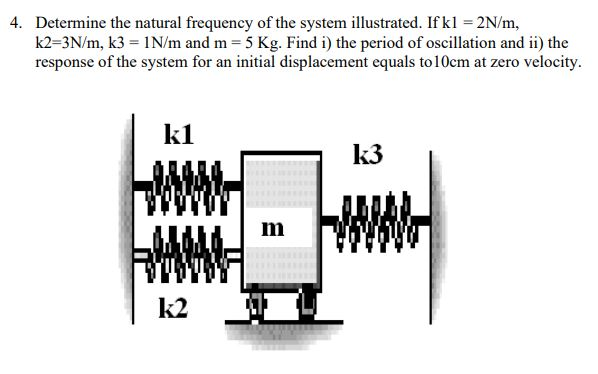Home / Expert Answers / Mechanical Engineering / 4-determine-the-natural-frequency-of-the-system-illustrated-if-k1-2n-m-k2-3n-m-k3-1n-m-and-pa336

# (Solved): 4. Determine the natural frequency of the system illustrated. If k1=2N/m, k2=3N/m,k3=1N/m and ...4. Determine the natural frequency of the system illustrated. If , and . Find i) the period of oscillation and ii) the response of the system for an initial displacement equals to at zero velocity.

We have an Answer from Expert